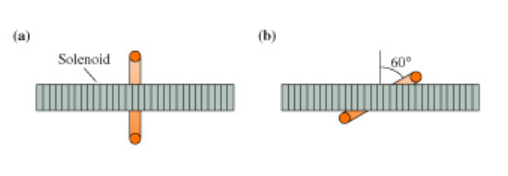# Problem: The 2.0-cm-diameter solenoid in the figure passes through the center of a 6.0-cm-diameter loop. The magnetic field inside the solenoid is 0.20What is the magnetic flux through the loop when it is tilted at a 60° angle?Express your answer using two significant figures.

###### FREE Expert Solution

Magnetic flux through a surface:

r = D/2 = (2.0 cm/2)(1m/100cm) = 0.01 m

89% (311 ratings)###### Problem Details

The 2.0-cm-diameter solenoid in the figure passes through the center of a 6.0-cm-diameter loop. The magnetic field inside the solenoid is 0.20

What is the magnetic flux through the loop when it is tilted at a 60° angle?